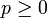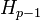# Dual universal coefficient theorem for group cohomology

Jump to: navigation, search

## Statement

### For coefficients in an abelian group

Suppose$G$ is a group and$M$ is an abelian group. The dual universal coefficients theorem relates the homology groups for trivial group action of$G$ on$\mathbb{Z}$ and the cohomology groups for trivial group action of$G$ on$M$ as follows:

First, for any$p\ge 0$, there is a natural short exact sequence of abelian groups:$0 \to \operatorname{Ext}(H_{p-1}(G;\mathbb{Z}),M) \to H^p(G;M) \to \operatorname{Hom}(H_p(G;\mathbb{Z}),M) \to 0$

Second, the sequence splits (not necessarily naturally), and we get:$H^p(G;M) \cong \operatorname{Hom}(H_p(G;\mathbb{Z}),M) \oplus \operatorname{Ext}(H_{p-1}(G;\mathbb{Z}),M)$

### For coefficients in the integers

This is the special case where$M = \mathbb{Z}$. In this case, we case:$H^p(G;\mathbb{Z}) \cong \operatorname{Hom}(H_p(G;\mathbb{Z}),\mathbb{Z}) \oplus \operatorname{Ext}(H_{p-1}(G;\mathbb{Z}),\mathbb{Z})$

### Typical case of finitely generated abelian groups

Suppose$H_p(G;\mathbb{Z}) \cong \mathbb{Z}^{r_p} \oplus T_p$ for some finite group$T_p$ and$H_{p-1}(G;\mathbb{Z}) \cong \mathbb{Z}^{r_{p-1}} \oplus T_{p-1}$ for some finite group$T_{p-1}$. Suppose further that:$T_p \cong \mathbb{Z}/a_1\mathbb{Z} \oplus \dots \mathbb{Z}/a_s\mathbb{Z}$

and$T_{p-1} \cong \mathbb{Z}/b_1\mathbb{Z} \oplus \dots \mathbb{Z}/b_t\mathbb{Z}$

Then we have:$H^p(G;M) \cong M^{r_p} \oplus \operatorname{Hom}(T_p,M) \oplus \operatorname{Ext}(T_{p-1},M)$

where we further have:$\operatorname{Hom}(T_p,M) \cong \bigoplus_{1 \le i \le s} \operatorname{Ann}_M(a_i)$

where$\operatorname{Ann}_M(a_i) = \{ x \in M \mid a_ix = 0 \}$, i.e., the$a_i$-torsion of$M$.

Also:$\operatorname{Ext}(T_{p-1},M) \cong \bigoplus_{1 \le i \le t} M/b_iM$

Thus, we get overall that:$H^p(G;M) \cong M^{r_p} \oplus \bigoplus_{1 \le i \le s} \operatorname{Ann}_M(a_i) \oplus \bigoplus_{1 \le i \le t} M/b_iM$

Finally, suppose:$M \cong \mathbb{Z}^w \oplus \mathbb{Z}/c_1\mathbb{Z} \oplus \dots \mathbb{Z}/c_u\mathbb{Z}$

In this case, the expressions simplify further:$\operatorname{Hom}(T_p,M) \cong \bigoplus_{1 \le i \le s, 1 \le j \le u} \mathbb{Z}/\operatorname{gcd}(a_i,c_j)\mathbb{Z}$

and:$\operatorname{Ext}(T_{p-1},M) \cong T_{p-1}^w \oplus \bigoplus_{1 \le i \le t, 1 \le j \le u} \mathbb{Z}/\operatorname{gcd}(b_i,c_j)\mathbb{Z}$

### Typical case of finitely generated abelian groups and coefficients in the integers

Suppose$H_p(G;\mathbb{Z}) \cong \mathbb{Z}^{r_p} \oplus T_p$ for some finite group$T_p$ and$H_{p-1}(G;\mathbb{Z}) \cong \mathbb{Z}^{r_{p-1}} \oplus T_{p-1}$ for some finite group$T_{p-1}$. Then:$H^p(G;\mathbb{Z}) \cong \mathbb{Z}^{r_p} \oplus T_{p-1}$

In other words, we pick the torsion-free part of$H_p$ and the torsion part of$H_{p-1}$ (roughly speaking).